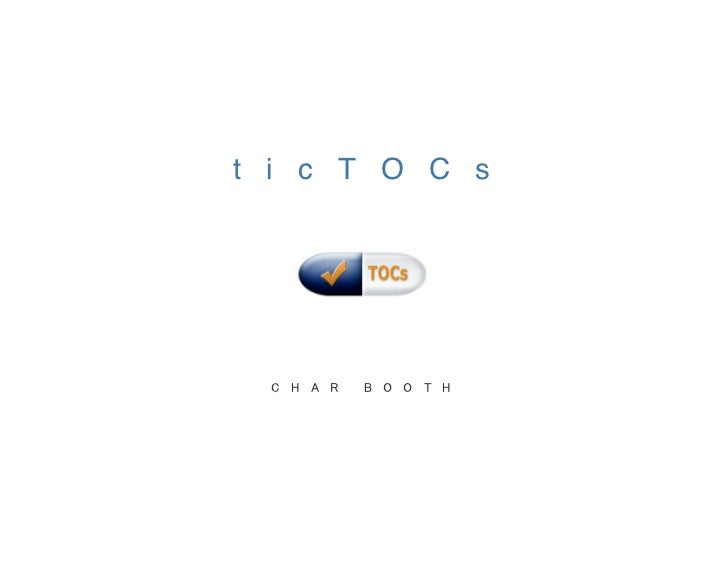Successfully reported this slideshow.Upcoming SlideShare
×

ticTOCs

906 views

Published on

This presentation was part of the UC Berkeley Library Emerging Technology Lightning Talks, an event held in early September 2009. A series of volunteer presentations about 6 minutes in length covered various uses of social/dynamic library technologies. We asked the audience to indicate their interest in further training on each subject (Omeka, ticTOCs, and Netvibes won the day).

Published in: Education, Technology
• Full Name
Comment goes here.

Are you sure you want to Yes No• Be the first to comment

• Be the first to like this

ticTOCs

1. 1. t i c T O C s
2. 2. { c u r r e n t a w a r e n e s s } {
3. 3. { c u r r e n t a w a r e n e s s } {{{
4. 4. { c u r r e n t a w a r e n e s s } { { { { { { { { { { { { { { { { { { { {
5. 5. s e a r c h r e v i e w s a v e
6. 6. d i r e c t e x p o r t t o R e f W o r k s
7. 7. subscribe to TOC feeds via many services
8. 8. v i e w l i c e n s e d c o n t e n t s u b s c r i b e t o a l e r t s
9. 9. e m b e d T O C s a n d a b s t r a c t s i n O P A C s
10. 10. S E A R C H R E V I E W S A V E E X P O R T A C C E S S E M B E D 1 2 , 6 0 0 + T O C R S S F E E D S
11. 11. { w w w . t i c t o c s . a c . u k }